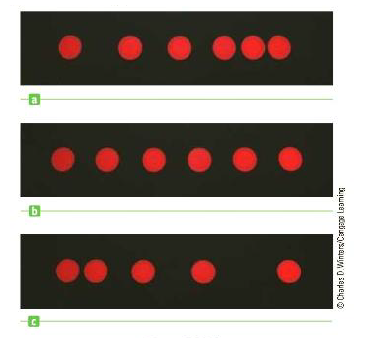Chapter 2, Problem 6CQ

Chapter
Section
Textbook Problem

Figure CQ2.6 shows strobe photographs taken of a disk moving from left to right under different conditions. The time interval between images is constant. Taking the direction to the right, to be positive, describe the motion of the disk in each case. For which case is (a) the acceleration positive? (b) the acceleration negative? (c) the velocity constant?Figure CQ2.6

(a)

To determine
The graph represents positive acceleration.

Explanation

Acceleration is change in velocity with time and the velocity is change in position with time.

The distance covered by an object is approximately twice in the second interval of time. In the next interval, the position varies three times more than the initial rate. In the final interval, the distance is four times the initial interval. The velocity of the object is increasing with time since the change in the position is positive. Therefore, the acceleration is positive in graph (c). Thus, the acceleration is positive in graph (c).

The distance covered by the object is approximately equal in the first three intervals of time...

(b)

To determine
The graph represents negative acceleration.

(c)

To determine
The graph represents constant velocity.

Still sussing out bartleby?

Check out a sample textbook solution.

See a sample solution

The Solution to Your Study Problems

Bartleby provides explanations to thousands of textbook problems written by our experts, many with advanced degrees!

Get Started